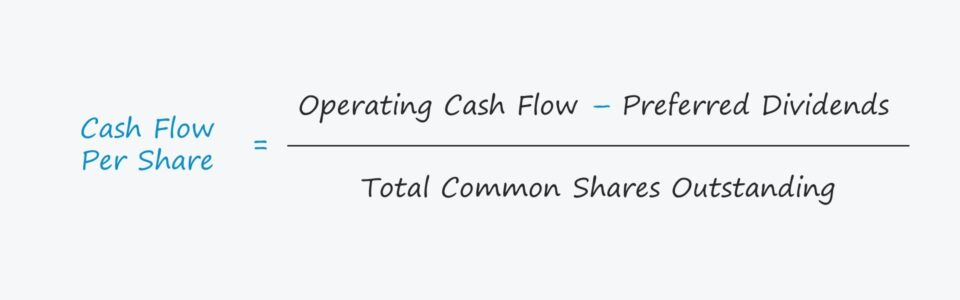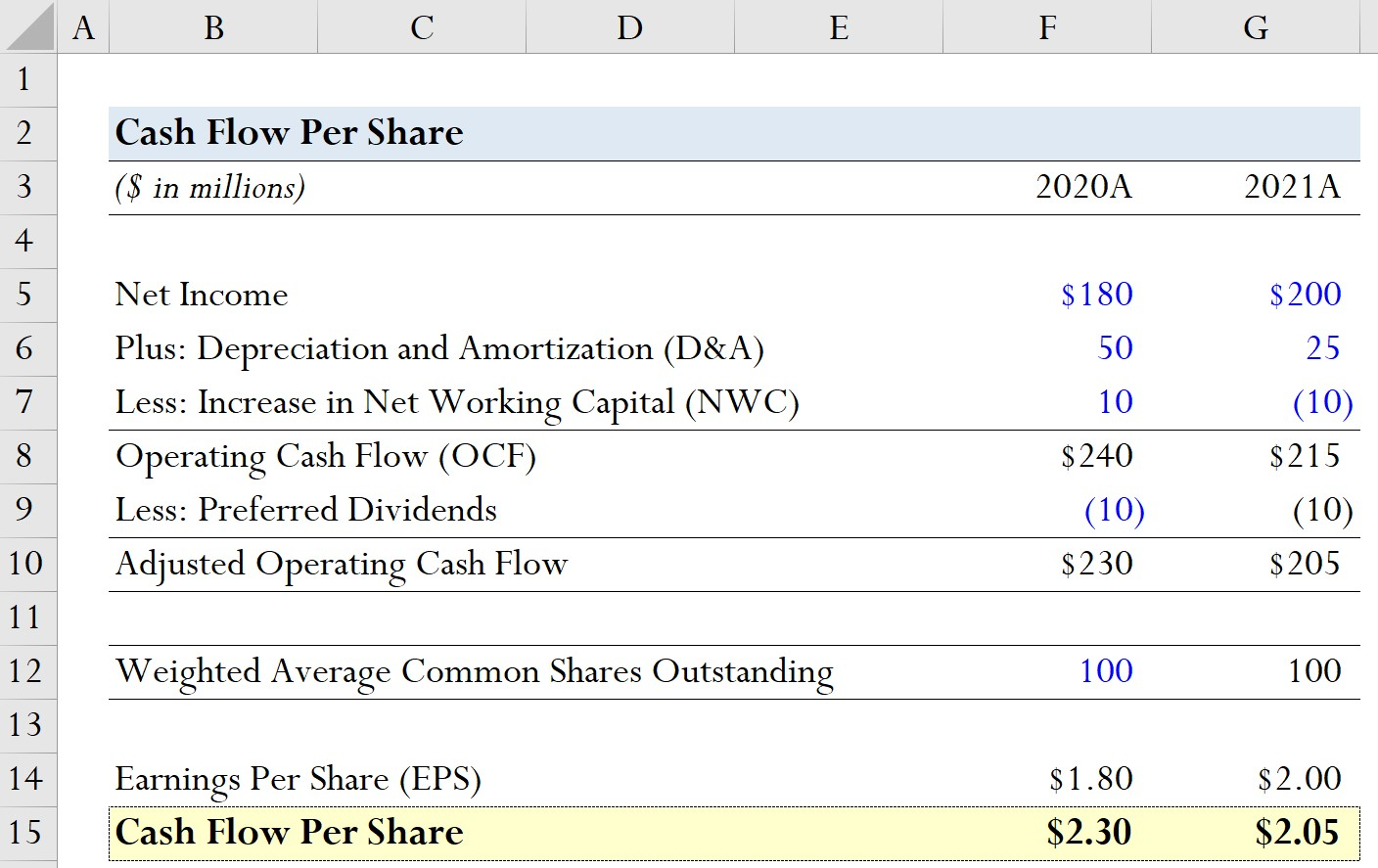Welcome to Wall Street Prep! Use code at checkout for 15% off.# Cash Flow Per Share

Guide to Understanding the Cash Flow Per Share## How to Calculate Cash Flow Per Share (Step-by-Step)

In order to calculate a company’s cash flow per share, its operating cash flow (OCF) is first adjusted by any preferred dividend issuances and then divided by its total common shares outstanding.

• Operating Cash Flow (OCF) → OCF measures the net cash generated from a company’s core operations within a specified period. The operating cash flow (OCF) metric, or cash flow from operations, is meant to represent the cash flows generated from the core, recurring operations of a company.
• Preferred Dividends → Dividend issuances paid to the owners of a company’s preferred stock, which hold precedence over common shareholders.
• Total Common Shares Outstanding → The total weighted average number of common shares outstanding, i.e. each share is weighted by the proportion of the given fiscal year in which the share was “outstanding”.

## Cash Flow Per Share Formula

The formula for calculating the cash flow per share metric is as follows.

Cash Flow Per Share = (Operating Cash Flows Preferred Dividends) ÷ Total Number of Common Shares Outstanding

However, there are numerous variations of the metric wherein free cash flow (FCF) metrics such as free cash flow to equity (FCFE) are used instead of operating cash flow (OCF).

Companies with more operating cash flows are better positioned to reinvest back into their operations, which indirectly benefits shareholders via share price appreciation, if publicly traded. The company could also repurchase shares or issue dividends to common shareholders, which is a form of direct compensation by either reducing dilution or via cash payments.

## Cash Flow Per Share vs. Earnings Per Share (EPS)

The earnings per share (EPS) formula divides net income by the total number of common shares outstanding, most often on a diluted basis.

Earnings Per Share (EPS) = Net Income ÷ Total Number of Diluted Common Shares Outstanding

One notable use-case of the cash flow per share metric is that it can be used to support a company’s earnings per share (EPS) growth, i.e. to confirm that EPS increased year-over-year (YoY) due to greater profitability and cash flows rather than accounting tricks (or even fraud).

The distinction between the two metrics is tied to the company’s investing and financing activities.

• Capital Structure: The effect of capital structure decisions and non-operating items on net income is one of the limitations to earnings per share (EPS) that makes it vulnerable to earnings management.
• Net Income: Unlike net income, the cash flow from operations metric is far more difficult for management to “doctor” and intentionally misguide investors, as there are fewer discretionary decisions. The accrual-based net income metric is subject to discretionary decisions by management with regard to accounting policies, e.g. the useful life assumption on fixed assets (PP&E). In contrast, the operating cash flow (OCF) of a company, while still imperfect, adjusts for non-cash items such as depreciation and amortization – which causes the value to be more reliable.

## Cash Flow Per Share Calculator – Excel Template

We’ll now move on to a modeling exercise, which you can access by filling out the form below.Submitting...

## Cash Flow Per Share Calculation Example

Suppose a company had the following historical financial data from the past two fiscal years.

Model Assumptions
(\$ in millions) 2020A 2021A
Net Income \$180 million \$200 million
Plus: Depreciation and Amortization (D&A) \$50 million \$25 million
Less: Increase in Net Working Capital (NWC) \$10 million (\$10 million)

Using these model assumptions, we can add D&A and subtract the increase in NWC to calculate the operating cash flow for each period.

• 2020A
• Operating Cash Flow (OCF) = \$180 million + \$50 million + \$10 million = \$240 million
• 2021A
• Operating Cash Flow (OCF) = \$200 million + \$25 million – \$10 million = \$215 million

From the OCF calculations, we can see that the company’s OCF has declined by \$15 million year-over-year, so it would be reasonable to assume that cash flow per share will also be lower in 2021.

In the next step, we’ll assume that our company’s preferred dividend issuance amounted to \$10 million in both periods.

• 2020A
• Adjusted Operating Cash Flow = \$240 million – \$10 million = \$230 million
• 2021A
• Adjusted Operating Cash Flow = \$215 million – \$10 million = \$205 million

As for our hypothetical company’s share count, we’ll assume the weighted average common shares outstanding stays constant at 100 million in both years.

• Weighted Average Common Shares Outstanding = 100 million

In order to see where the cash flow per share metric can be most useful, we’ll also calculate the earnings per share (EPS) of our company.

• 2020A
• Earnings Per Share (EPS) = \$180 million ÷ 100 million = \$1.80
• 2021A
• Earnings Per Share (EPS) = \$200 million ÷ 100 million = \$2.00

From 2020 to 2021, our company’s EPS grew from \$1.80 to \$2.00, an increase of \$0.20.

In the final part of our modeling exercise, we’ll calculate the cash flow per share for each period.

• 2020A
• Cash Flow Per Share = \$230 million ÷ 100 million = \$2.30
• 2021A
• Cash Flow Per Share = \$205 million ÷ 100 million = \$2.05

Therefore, by calculating cash flow per share, we’ve identified that the company’s positive EPS growth is questionable and must further investigate to determine the real driver behind the growth.Step-by-Step Online Course

### Everything You Need To Master Financial Modeling

Enroll in The Premium Package: Learn Financial Statement Modeling, DCF, M&A, LBO and Comps. The same training program used at top investment banks.